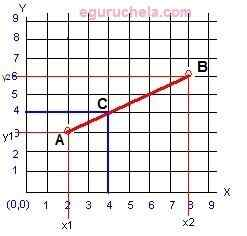### Linear Interpolation Value Calculation

Linear interpolation calculator finds y-axis value for given (x1 , y1) (x2 , y2) coordinates and targeted x-axis value. Linear interpolation is a method of curve fitting using linear polynomials. It is useful in numerical analysis, computer graphics. For given the two (x & y coordinates) points, the line is the linear interpolant between the points. The value of y at given x may be found by linear interpolation.Enter the first co-ordinates :      x1  y1 Enter the second co-ordinates : x2   y2 Enter the Target x : Interpolated y value: